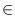Next: Graph Drawing Algorithms ( Up: Graph Algorithms Previous: Euler Tours ( euler_tour   Contents   Index

# Algorithms for Planar Graphs ( plane_graph_alg )

 node ST_NUMBERING(const graph& G, node_array& stnum, list& stlist, edge e_st = nil) ST_NUMBERING computes an st-numbering of G. If e_st is nil then t is set to some arbitrary node of G. The node s is set to a neighbor of t and is returned. If e_st is not nil then s is set to the source of e_st and t is set to its target. The nodes of G are numbered such that s has number 1, t has number n, and every node v different from s and t has a smaller and a larger numbered neighbor. The ordered list of nodes is returned in stlist. If G has no nodes then nil is returned and if G has exactly one node then this node is returned and given number one. Precondition G is biconnected. bool PLANAR(graph& , bool embed=false) PLANAR takes as input a directed graph G(V, E) and performs a planarity test for it. G must not contain self-loops. If the second argument embed has value true and G is a planar graph it is transformed into a planar map (a combinatorial embedding such that the edges in all adjacency lists are in clockwise ordering). PLANAR returns true if G is planar and false otherwise. The algorithm () has running time O(| V| + | E|). bool PLANAR(graph& G, list& el, bool embed=false) PLANAR takes as input a directed graph G(V, E) and performs a planarity test for G. PLANAR returns true if G is planar and false otherwise. If G is not planar a Kuratowski-Subgraph is computed and returned in el. bool CHECK_KURATOWSKI(const graph& G, const list& el) returns true if all edges in el are edges of G and if the edges in el form a Kuratowski subgraph of G, returns false otherwise. Writes diagnostic output to cerr. int KURATOWSKI(graph& G, list& V, list& E, node_array& deg) KURATOWSKI computes a Kuratowski subdivision K of G as follows. V is the list of all nodes and subdivision points of K. For all vV the degree deg[v] is equal to 2 for subdivision points, 4 for all other nodes if K is a K5, and -3 (+3) for the nodes of the left (right) side if K is a K3, 3. E is the list of all edges in the Kuratowski subdivision. list TRIANGULATE_PLANAR_MAP(graph& G) TRIANGULATE_PLANAR_MAP takes a directed graph G representing a planar map. It triangulates the faces of G by inserting additional edges. The list of inserted edges is returned. Precondition G must be connected. The algorithm () has running time O(| V| + | E|). void FIVE_COLOR(graph& G, node_array& C) colors the nodes of G using 5 colors, more precisely, computes for every node v a color C[v]{0,..., 4}, such that C[source(e)]! = C[target(e)] for every edge e. Precondition G is planar. Remark: works also for many (sparse ?) non-planar graph. void INDEPENDENT_SET(const graph& G, list& I) determines an independent set of nodes I in G. Every node in I has degree at most 9. If G is planar and has no parallel edges then I contains at least n/6 nodes. bool Is_CCW_Ordered(const graph& G, const node_array& x, const node_array& y) checks whether the cyclic adjacency list of any node v agrees with the counter-clockwise ordering of the neighbors of v around v defined by their geometric positions. bool SORT_EDGES(graph& G, const node_array& x, const node_array& y) reorders all adjacency lists such the cyclic adjacency list of any node v agrees with the counter-clockwise order of v's neighbors around v defined by their geometric positions. The function returns true if G is a plane map after the call. bool Is_CCW_Ordered(const graph& G, const edge_array& dx, const edge_array& dy) checks whether the cyclic adjacency list of any node v agrees with the counter-clockwise ordering of the neighbors of v around v. The direction of edge e is given by the vector (dx(e), dy(e)). bool SORT_EDGES(graph& G, const edge_array& dx, const edge_array& dy) reorders all adjacency lists such the cyclic adjacency list of any node v agrees with the counter-clockwise order of v's neighbors around v. The direction of edge e is given by the vector (dx(e), dy(e)). The function returns true if G is a plane map after the call.Next: Graph Drawing Algorithms ( Up: Graph Algorithms Previous: Euler Tours ( euler_tour   Contents   Index